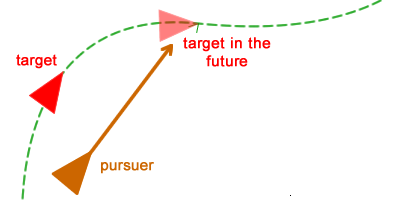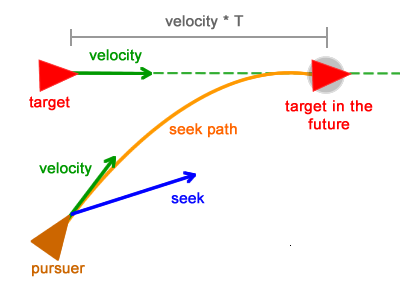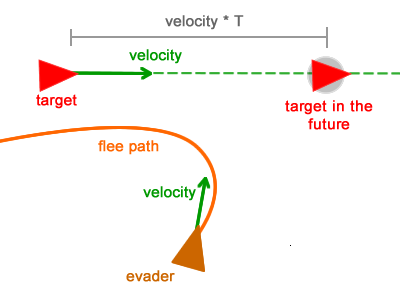# Steering Behaviors: Pursuit and Evade 追逐和躲避`position = position + velocity`

`position = position + velocity * T`

```public function pursuit(t :Boid) :Vector3D {
T :int  = 3;
futurePosition :Vector3D = t.position + t.velocity * T;
return seek(futurePosition);
}```

```public function update() :void {
steering = pursuit(target)
steering = truncate (steering, max_force)
steering = steering / mass
velocity = truncate (velocity + steering , max_speed)
position = position + velocity
}````T = distanceBetweenTargetAndPursuer / MAX_VELOCITY`

```public function pursuit(t :Boid) :Vector3D {
var distance :Vector3D = t.position - position;
var T :int = distance.length / MAX_VELOCITY;
futurePosition :Vector3D = t.position + t.velocity * T;
return seek(futurePosition);
}```

he evade behavior is the opposite of the pursuit behavior. Instead of seeking the target’s future position, in the evade behavior the character will flee that position:```public function evade(t :Boid) :Vector3D {
var distance :Vector3D = t.position - position;